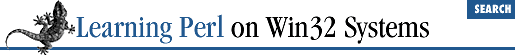home | O'Reilly's CD bookshelfs | FreeBSD | Linux | Cisco | Cisco Exam

### 8.4 Arguments

Although subroutines that have one specific action are useful, a whole new level of usefulness becomes available when you can pass arguments to a subroutine. In Perl, the subroutine invocation (with the ampersand and the subroutine name) is followed by a list within parentheses, causing the list to be automatically assigned to a special variable named ``` @_``` for the duration of the subroutine. The subroutine can access this variable to determine the number of arguments and the value of those arguments. For example:

```sub say_hello_to {
print "hello, \$_!\n"; # first parameter is target
}```

Here, we see a reference to ``` \$_``` , which is the first element of the ``` @_``` array. Special note: although similar in appearance, the ``` \$_``` value (the first element of the ``` @_``` array) has nothing whatsoever to do with the ``` \$_``` variable (a scalar variable of its own). Don't confuse them! The code seems to say hello to whomever we pass as the first parameter. As a result, we can invoke it like this:

```say_hello_to("world");                    # gives hello, world!
\$x = "somebody";
say_hello_to(\$x);                         # gives hello, somebody!
say_hello_to("me") + say_hello_to("you"); # and me and you```

Note that in the last line, the return values weren't really used. But in evaluating the sum, Perl has to evaluate all of its parts, so the subroutine was invoked twice.

Here's an example using more than one parameter:

```sub say {
print "\$_, \$_!\n";
}

say("hello","world");         # hello world, once again
say("goodbye","cruel world"); # silent movie lament```

Excess parameters are ignored: if you never look at ``` \$_``` , Perl doesn't care. And insufficient parameters are also ignored; you simply get ``` undef``` if you look beyond the end of the ``` @_``` array, as with any other array.

The ``` @_``` variable is private to the subroutine; if there's a global value for ``` @_``` , it is saved away before the subroutine is invoked and restored to its previous value upon return from the subroutine. This also means that a subroutine can pass arguments to another subroutine without fear of losing its own ``` @_``` variable; the nested subroutine invocation gets its own ``` @_``` in the same way.

Let's revisit that "add a and b" routine from the previous section. Here's a subroutine that adds any two values (specifically, the two values passed to the subroutine as parameters):

```sub add_two {
\$_ + \$_;
}
\$c = add_two(5,6);  # \$c gets 11```

Now let's generalize this subroutine. What if we had 3, 4, or 100 values to add together? We could do it with a loop, as shown:

```sub add {
\$sum = 0;          # initialize the sum
foreach \$_ (@_) {
\$sum += \$_;    # add each element
}
return \$sum;       # the sum of all elements
}
\$a = add(4,5,6);       # adds 4+5+6 = 15, and assigns to \$a
What if we had a variable named ``` \$sum``` when we called ``` add``` ? We just clobbered it. In the next section, we see how to avoid this situation.8.3 Return Values8.5 Private Variables in Functions